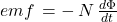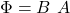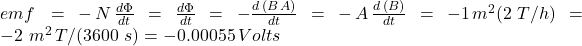## A loop of wire with cross-sectional area 1 m2 is inserted into a uniform magnetic field with initial strength 1 T. The field is parallel to

Question

A loop of wire with cross-sectional area 1 m2 is inserted into a uniform magnetic field with initial strength 1 T. The field is parallel to the axis of the loop. The field begins to grow with time at a rate of 2 Teslas per hour. What is the magnitude of the induced EMF in the loop of wire

in progress 0
6 months 2021-08-18T07:35:31+00:00 1 Answers 2 views 0

## Answers ( )

The magnitude of the EMF is 0.00055  volts

Explanation:

The induced EMF is proportional to the change in magnetic flux based on Faraday’s law:Since in our case there is only one loop of wire, then N=1 and we get:We need to express the magnetic flux given the geometry of the problem;where A is the area of the coil that remains unchanged with time, and B is the magnetic field that does change with time. Therefore the equation for the EMF becomes: